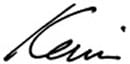# How to Verify The Quantity of Your Just Delivered Bulk Landscaping MaterialsWhen you make purchases from a wholesale landscaping company, do you ever wonder if you are truly getting what you paid for?

For example, if you order 10 yards of topsoil, how can you determine that you received 10 yards, not 5 yards? Well, I wrote this article to solve that question.

### CALCULATING THE VOLUME OF A CONE

In my experience, most bulk materials are delivered in tandem trucks. And when the materials are dumped, these piles usually (somewhat) resemble cones.

We have a neat little cone volume formula, we can take some measurements to verify that we did not get shortchanged.

• Determine the area of a base;
• Determine the height;
• Calculate the volume;

Please keep in mind that calculated volume is an approximate since measurements for the circumference (distance around the base) and the height will all be imperfect. However, it will give you a good idea if you received 7 yards vs. 10 yards that you paid for.

#### STEP 1 – CALCULATE THE AREA OF A BASE

In order to determine the base area, we need to obtain the radius of the dirt pile.

1. Since we can’t directly measure the radius without getting messy, we need to derive it by doing some calculations with a circumference.
2. Walk around the base of the material and take a measurement in liner feet. You can use a soft tape measure (like this), rolling tape (like this), plain string or even your feet.
3. Divide circumference by 6.28 to determine the diameter (D) of a base circle.
4. Then, divide the diameter by 2 to solve for the radius (R)
• Now that we have a value for radius, we can determine the total area of the base circle by using this formula:
For example, I walked around the base of my dirt pile and determined it to be 19.5 feet. Then I simply plugged in this number in to the equation

• Circumference = 19.5 feet
• Diameter = 19.5 feet / 6.28 = 3.11 feet
• Radius = 3.11 feet / 2 = 1.55 feet
• Area of a Base Circle = 3.14159 x (1.55 x 1.55)
• Area of a Base Circle = 7.57 sq feet

#### STEP 2 – CALCULATE THE HEIGHT

Now that we have the base area, all we need is the height of the dirt pile. I suppose you can climb to top of the dirt pile and stick a long piece of wood to measure the height, but there is a better way.

The image left is a simple math equation called Pythagorean Theorem. Before you roll your eyes, just know that all it says is that if you know 2 out of 3 variables (a, b, c), you can find a value for the unknown 3rd variable.

Now compare it to the image on the right. Anything look familiar?

That’s right. We already know the radius from STEP 1 so let’s assign that value to letter “b”.
For “c” or hypotenuse, take a steel tape measure (like this) from the peak of the dirt pile to the bottom.

Now that we know “b” and “c”, we can solve for “a” or height using the Pythagorean Theorem:

• a2 + b2 = c2 OR (a x a) + (b x b) = (c x c)
• (a x a) = (c x c) – (b x b)
• (a x a) = (height x height) – (radius x radius)
• a = SQRT [ (height x height) – (radius x radius)]

For example, I when I measured the hypotenuse, it came out to be 9.5 feet so let’s plug it into the equation and see what happens:

• c or hypotenuse = 9.5 feet
• b or radius = 3.11 feet
• a2 = (9.5 x 9.5) – (3.11 x 3.11)
• a2 = 90.25 – 9.67
• a2 = 80.58
• a = SQRT (80.58)
• h = 8.98 ft

So the height for my dirt pile is approximately 9 feet tall.

#### STEP 3 – CALCULATE VOLUME OF A CONE (FINALLY!)

Finally, we can use the Vcone formula to compute the approximate volume:

• Vcone = 1/3 × base area × height
• Vcone = 3.33 x 7.57 SQ FT x 9 FT
• Vcone = 226.8729 CU FT
• Vcone = 227.8729 / 27 = 8.4 CU YDS

So the approximate volume on the dirt pile is 8.4 CU YDS (or just “yards”) sounds about right (because I ordered 10 yards of dirt and it ended up being contaminated with black mulch).

Well, I hope you found this article to be useful when you order bulk landscaping materials for your project.

It would be great if you can signup for my newsletter so that I can alert you to my future AllThumbsDIY articles. The signup form is found on the upper right hand corner of your screen.

Thanks and good luck!